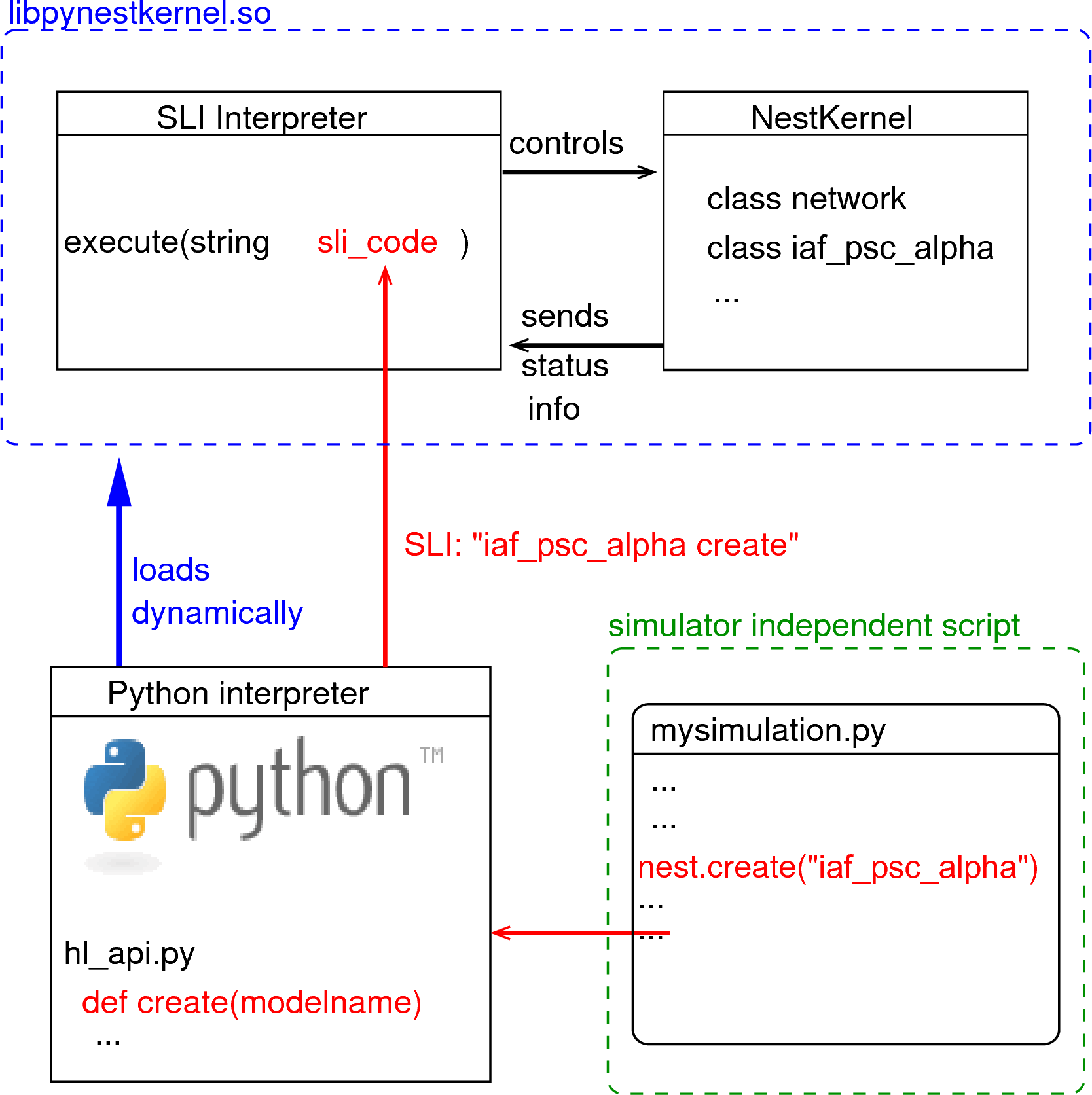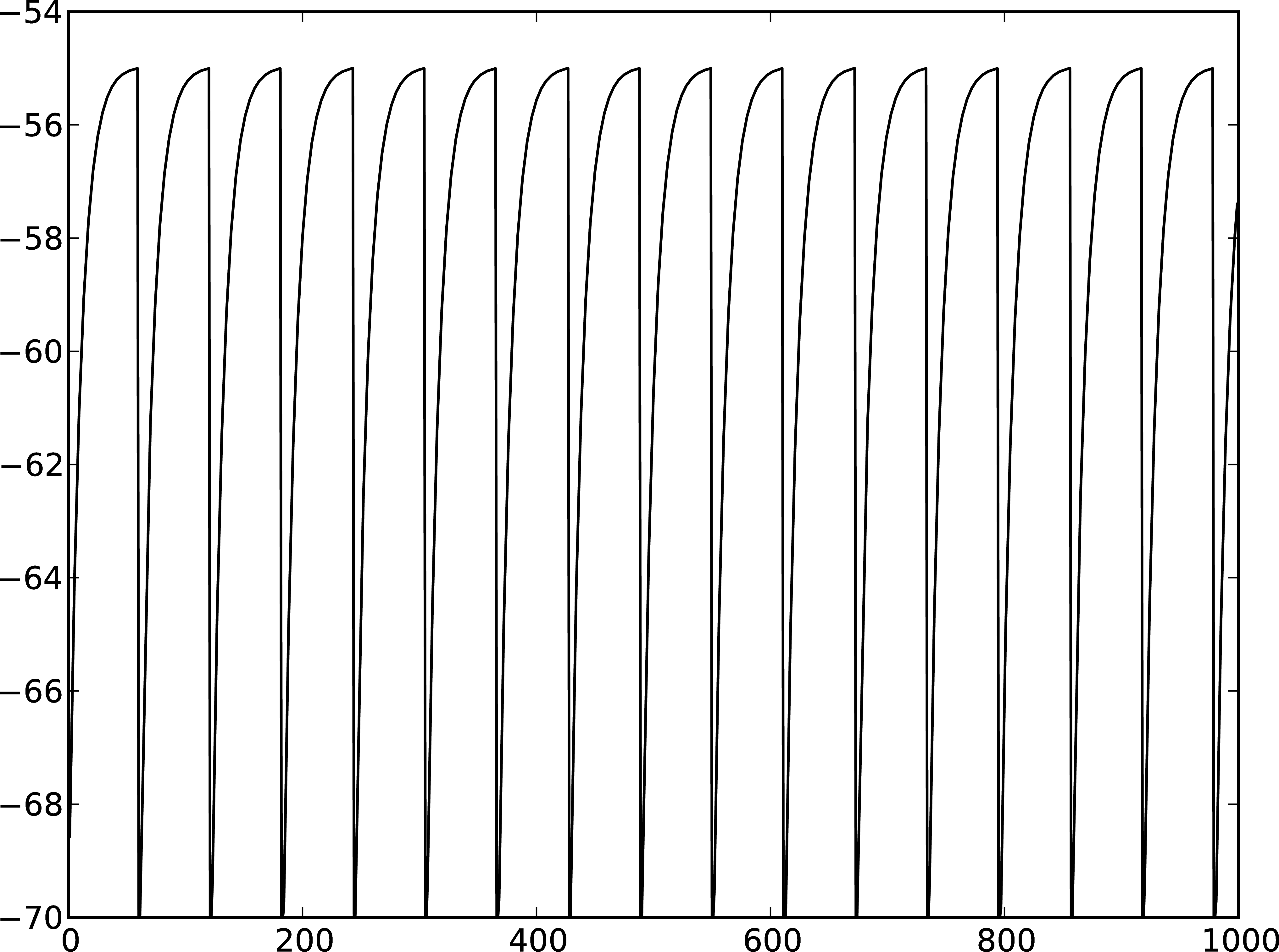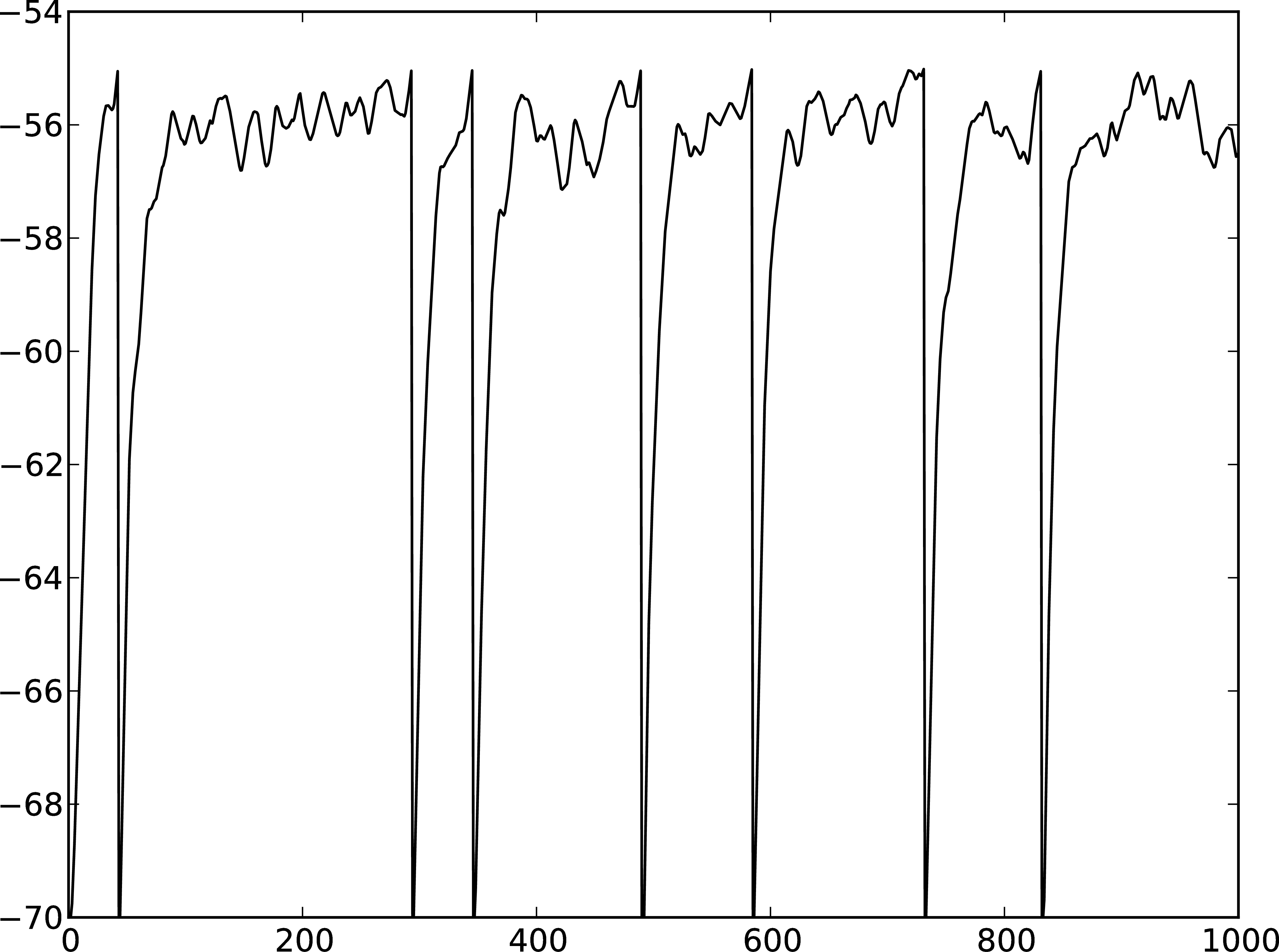# Part 1: Neurons and simple neural networks¶

## Introduction¶

In this section we cover the first steps in using PyNEST to simulate neuronal networks. When you have worked through this material, you will know how to:

• start PyNEST

• create neurons and stimulation or recording devices

• query and set their parameters

• connect them to each other or to devices

• simulate the network

• extract the data from recording devices

More advanced examples can be found at Example Networks, or have a look at at the source directory of your NEST installation in the subdirectory: `pynest/examples/`.

## PyNEST - an interface to the NEST simulator¶Figure 25 Python Interface Figure. The Python interpreter imports NEST as a module and dynamically loads the NEST simulator kernel (`pynestkernel.so`). The core functionality is defined in `hl_api.py`. A simulation script of the user (`mysimulation.py`) uses functions defined in this high-level API. These functions generate code in SLI (Simulation Language Interpreter), the native language of the interpreter of NEST. This interpreter, in turn, controls the NEST simulation kernel.

The NEural Simulation Tool (NEST: www.nest-initiative.org) 1 is designed for the simulation of large heterogeneous networks of point neurons. It is open source software released under the GPL licence. The simulator comes with an interface to Python 2. Figure 25 illustrates the interaction between the user’s simulation script (`mysimulation.py`) and the NEST simulator. Eppler et al. 3 contains a technically detailed description of the implementation of this interface and parts of this text are based on this reference. The simulation kernel is written in C++ to obtain the highest possible performance for the simulation.

You can use PyNEST interactively from the Python prompt or from within IPython/Jupyter. This is very helpful when you are exploring PyNEST, trying to learn a new functionality or debugging a routine. Once out of the exploratory mode, you will find it saves a lot of time to write your simulations in text files. These can in turn be run from the command line or from the Python or ipython prompt.

Whether working interactively, semi-interactively, or purely executing scripts, the first thing that needs to happen is importing NEST’s functionality into the Python interpreter.

```import nest
```

In case other Python packages are required, such as scikit-learn and SciPy, they need to be imported before importing NEST.

```import sklearn
import scipy

import nest
```

As with every other module for Python, the available functions can be prompted for.

```dir(nest)
```

One such command is `nest.Models()`, which will return a list of all the available models you can use. If you want to obtain more information about a particular command, you may use Python’s standard help system.

This will return the help text (docstring) explaining the use of this particular function. There is a help system within NEST as well. You can open the help pages in a browser using `nest.helpdesk()` and you can get the help page for a particular NEST object (like a synapse or neuron model) using `nest.help(object)`.

## Creating Nodes¶

A neural network in NEST consists of two basic element types: nodes and connections. Nodes are either neurons, devices or sub-networks. Devices are used to stimulate neurons or to record from them. Nodes can be arranged with spatial structure to build networks distributed in space - we will get to this later in the course. For now we will work with the default network structure of NEST.

New nodes are created with the command `Create`, which takes as arguments the model name of the desired node type, and optionally the number of nodes to be created and the initialising parameters. The function returns a `NodeCollection` of handles to the new nodes, which you can assign to a variable for later use. A `NodeCollection` is a compact representation of the node handles, which are integer numbers, called ids. Many PyNEST functions expect or return a `NodeCollection` (see command overview). Thus, it is easy to apply functions to large sets of nodes with a single function call.

After having imported NEST and Matplotlib 4, which we will use to display the results, we can start creating nodes. As a first example, we will create a neuron of type `iaf_psc_alpha`. This neuron is an integrate-and-fire neuron with alpha-shaped postsynaptic currents. The function returns a NodeCollection of the ids of all the created neurons, in this case only one, which we store in a variable called `neuron`.

```import matplotlib.pyplot as plt
import nest
neuron = nest.Create("iaf_psc_alpha")
```

We can now use the NodeCollection to access the properties of this neuron. Properties of nodes in NEST are generally accessed via Python dictionaries of key-value pairs of the form `{key: value}`. In order to see which properties a neuron has, you may ask it for its status.

```neuron.get()
```

This will print out the corresponding dictionary in the Python console. Many of these properties are not relevant for the dynamics of the neuron. To find out what the interesting properties are, look at the documentation of the model through the helpdesk. If you already know which properties you are interested in, you can specify a key, or a list of keys, as an optional argument to `get`:

```neuron.get("I_e")
neuron.get(["V_reset", "V_th"])
```

In the first case we query the value of the constant background current `I_e`; the result is given as a floating point element. In the second case, we query the values of the reset potential and threshold of the neuron, and receive the result as a dictionary . If `get` is called on a NodeCollection with more than one element, the returned dictionary will contain lists with the same number of elements as the number of nodes in the NodeCollection. If `get` is called with a specific key on a NodeCollection with several elements, a list the size of the NodeCollection will be returned.

To modify the properties in the dictionary, we use `set`. In the following example, the background current is set to 375.0pA, a value causing the neuron to spike periodically.

```neuron.set(I_e=375.0)
```

Note that we can set several properties at the same time by giving multiple comma separated key:value pairs in a dictionary. Also be aware that NEST is type sensitive - if a particular property is of type `double`, then you do need to explicitly write the decimal point:

```neuron.set({"I_e": 375})
```

will result in an error. This conveniently protects us from making integer division errors, which are hard to catch.

Another way of setting and getting parameters is to ask the NodeCollection directly

```neuron.I_e = 376.0
neuron.I_e
```

Next we create a `multimeter`, a device we can use to record the membrane voltage of a neuron over time. The property `record_from` expects a list of the names of the variables we would like to record. The variables exposed to the multimeter vary from model to model. For a specific model, you can check the names of the exposed variables by looking at the neuron’s property `recordables`.

```multimeter = nest.Create("multimeter")
multimeter.set(record_from=["V_m"])
```

We now create a `spike_recorder`, another device that records the spiking events produced by a neuron.

```spikerecorder = nest.Create("spike_recorder")
```

A short note on naming: here we have called the neuron `neuron`, the multimeter `multimeter` and so on. Of course, you can assign your created nodes to any variable names you like, but the script is easier to read if you choose names that reflect the concepts in your simulation.

## Connecting nodes with default connections¶

Now we know how to create individual nodes, we can start connecting them to form a small network.

```nest.Connect(multimeter, neuron)
nest.Connect(neuron, spikerecorder)
```Figure 26 Membrane potential of integrate-and-fire neuron with constant input current.

The order in which the arguments to `Connect` are specified reflects the flow of events: if the neuron spikes, it sends an event to the spike recorder. Conversely, the multimeter periodically sends requests to the neuron to ask for its membrane potential at that point in time. This can be regarded as a perfect electrode stuck into the neuron.

Now we have connected the network, we can start the simulation. We have to inform the simulation kernel how long the simulation is to run. Here we choose 1000ms.

```nest.Simulate(1000.0)
```

Congratulations, you have just simulated your first network in NEST!

## Extracting and plotting data from devices¶

After the simulation has finished, we can obtain the data recorded by the multimeter.

```dmm = multimeter.get()
Vms = dmm["events"]["V_m"]
ts = dmm["events"]["times"]
```

In the first line, we obtain a dictionary with status parameters for the `multimeter`. This dictionary contains an entry named `events` which holds the recorded data. It is itself a dictionary with the entries `V_m` and `times`, which we store separately in `Vms` and `ts`, in the second and third line, respectively. If you are having trouble imagining dictionaries of dictionaries and what you are extracting from where, try first just printing `dmm` to the screen to give you a better understanding of its structure, and then in the next step extract the dictionary `events`, and so on.

Now we are ready to display the data in a figure. To this end, we make use of `matplotlib` and the `pyplot` module.

```import matplotlib.pyplot as plt
plt.figure(1)
plt.plot(ts, Vms)
```

The second line opens a figure (with the number 1), and the third line actually produces the plot. You can’t see it yet because we have not used `plt.show()`. Before we do that, we proceed analogously to obtain and display the spikes from the spike recorder.

```dSD = spikerecorder.get("events")
evs = dSD["senders"]
ts = dSD["times"]
plt.figure(2)
plt.plot(ts, evs, ".")
plt.show()
```

Here we extract the events more concisely by sending the parameter name to `get`. This extracts the dictionary element with the key `events` rather than the whole status dictionary. The output should look like Figure 26 and Figure 27. If you want to execute this as a script, just paste all lines into a text file named, say, `one-neuron.py` . You can then run it from the command line by prefixing the file name with `python`, or from the Python or ipython prompt, by prefixing it with `run`.

It is possible to collect information of multiple neurons on a single multimeter. This does complicate retrieving the information: the data for each of the n neurons will be stored and returned in an interleaved fashion. Luckily Python provides us with a handy array operation to split the data easily: array slicing with a step (sometimes called stride). To explain this you have to adapt the model created in the previous part. Save your code under a new name, in the next section you will also work on this code. Create an extra neuron with the background current given a different value:

```neuron2 = nest.Create("iaf_psc_alpha")
neuron2.set({"I_e": 370.0})
```

now connect this newly created neuron to the multimeter:

```nest.Connect(multimeter, neuron2)
```

Run the simulation and plot the results, they will look incorrect. To fix this you must plot the two neuron traces separately. Replace the code that extracts the events from the `multimeter` with the following lines.

```plt.figure(2)
Vms1 = dmm["events"]["V_m"][::2] # start at index 0: till the end: each second entry
ts1 = dmm["events"]["times"][::2]
plt.plot(ts1, Vms1)
Vms2 = dmm["events"]["V_m"][1::2] # start at index 1: till the end: each second entry
ts2 = dmm["events"]["times"][1::2]
plt.plot(ts2, Vms2)
```

Additional information can be found at http://docs.scipy.org/doc/numpy-1.10.0/reference/arrays.indexing.html.

## Connecting nodes with specific connections¶

A commonly used model of neural activity is the Poisson process. We now adapt the previous example so that the neuron receives 2 Poisson spike trains, one excitatory and the other inhibitory. Hence, we need a new device, the `poisson_generator`. After creating the neurons, we create these two generators and set their rates to 80000Hz and 15000Hz, respectively.

```noise_ex = nest.Create("poisson_generator")
noise_in = nest.Create("poisson_generator")
noise_ex.set(rate=80000.0)
noise_in.set(rate=15000.0)
```

Additionally, the constant input current should be set to 0:

```neuron.set(I_e=0.0)
```

Each event of the excitatory generator should produce a postsynaptic current of 1.2pA amplitude, an inhibitory event of -2.0pA. The synaptic weights can be defined in a dictionary, which is passed to the `Connect` function using the keyword `syn_spec` (synapse specifications). In general all parameters determining the synapse can be specified in the synapse dictionary, such as `"weight"`, `"delay"`, the synaptic model (`"synapse_model"`) and parameters specific to the synaptic model.

```syn_dict_ex = {"weight": 1.2}
syn_dict_in = {"weight": -2.0}
nest.Connect(noise_ex, neuron, syn_spec=syn_dict_ex)
nest.Connect(noise_in, neuron, syn_spec=syn_dict_in)
```Figure 28 Membrane potential of integrate-and-fire neuron with Poisson noise as input.

The rest of the code remains as before. You should see a membrane potential as in Figure 28 and Figure 29.

In the next part of the introduction (Part 2: Populations of neurons) we will look at more methods for connecting many neurons at once.

## Two connected neurons¶Figure 30 Postsynaptic potentials in neuron2 evoked by the spikes of neuron1

There is no additional magic involved in connecting neurons. To demonstrate this, we start from our original example of one neuron with a constant input current, and add a second neuron.

```import nest
neuron1 = nest.Create("iaf_psc_alpha")
neuron1.set(I_e=376.0)
neuron2 = nest.Create("iaf_psc_alpha")
multimeter = nest.Create("multimeter")
multimeter.set(record_from=["V_m"])
```

We now connect `neuron1` to `neuron2`, and record the membrane potential from `neuron2` so we can observe the postsynaptic potentials caused by the spikes of `neuron1`.

```nest.Connect(neuron1, neuron2, syn_spec = {"weight":20.0})
nest.Connect(multimeter, neuron2)
```

Here the default delay of 1ms was used. If the delay is specified in addition to the weight, the following shortcut is available:

```nest.Connect(neuron1, neuron2, syn_spec={"weight":20.0, "delay":1.0})
```

If you simulate the network and plot the membrane potential as before, you should then see the postsynaptic potentials of `neuron2` evoked by the spikes of `neuron1` as in Figure 30.

## Command overview¶

These are the functions we introduced for the examples in this handout; the following sections of this introduction will add more.

See the Getting Help Section

### Nodes¶

• `Create(model, n=1, params=None)`

Create `n` instances of type `model`. Parameters for the new nodes can be given as `params`, which can be any of the following:

• A dictionary with either single values or lists of size n. The single values will be applied to all nodes, while the lists will be distributed across the nodes. Both single values and lists can be given at the same time.

• A list with n dictionaries, one dictionary for each node.

If omitted, the `model`’s defaults are used.

• `get(*params, **kwargs)`

Return a dictionary with parameter values for the NodeCollection it is called on. If `params` is a single string, a list of values is returned instead. `params` may also be a list of strings, in which case the returned dictionary contains lists of requested values.

• `set(params=None, **kwargs)`

Set the parameters on the NodeCollection to `params`, which may be a single dictionary (with lists or single values as parameters), or a list of dictionaries of the same size as the NodeCollection. If `kwargs` is given, it has to be names and values of an attribute as keyword=argument pairs. The values can be single values or list of the same size as the NodeCollection.

### Connections¶

This is an abbreviated version of the documentation for the `Connect` function, please see NEST’s online help for the full version and Connection Management for an introduction and examples.

• `Connect(pre, post, conn_spec=None, syn_spec=None, return_synapsecollection=False)`

Connect pre neurons to post neurons. Neurons in pre and post are connected using the specified connectivity (`"all_to_all"` by default) and synapse type (`"static_synapse"` by default). Details depend on the connectivity rule. `pre` - presynaptic neurons, given as a NodeCollection of node IDs `post` - presynaptic neurons, given as a NodeCollection of node IDs `conn_spec` - name or dictionary specifying connectivity rule, see below `syn_spec` - name or dictionary specifying synapses, see below.

#### Connectivity¶

Connectivity is either specified as a string containing the name of a connectivity rule (default: `"all_to_all"`) or as a dictionary specifying the rule and rule-specific parameters (e.g. `"indegree"`), which must be given. In addition switches allowing self-connections (`"allow_autapses"`, default: `True`) and multiple connections between a pair of neurons (`"allow_multapses"`, default: `True`) can be contained in the dictionary.

#### Synapse¶

The synapse model and its properties can be inserted either as a string describing one synapse model (synapse models are listed in the synapsedict) or as a dictionary. If no synapse model is specified the default model `"static_synapse"` will be used. Available keys in the synapse dictionary are `"synapse_model"`, `"weight"`, `"delay"`, `"receptor_type"` and parameters specific to the chosen synapse model. All parameters are optional and if not specified will use the default values determined by the current synapse model. `"synapse_model"` determines the synapse type, taken from pre-defined synapse types in NEST or manually specified synapses created via `CopyModel()`. All other parameters can be scalars or distributions. In the case of scalar parameters, all keys take doubles except for `"receptor_type"` which has to be initialised with an integer. Distributed parameters are initialised with a Parameter with distribution-specific arguments (such as `"mean"` and `"std"`).

### Simulation control¶

• `Simulate(t)`

Simulate the network for `t` milliseconds.